# Area and Perimeter Worksheets Grade 6

Please check explanation and answers of Area and Perimeter Worksheets Grade 6 at the end of the questions.Q.1 Find the perimeter of a rectangle in which: length = 9 cm and breadth = 8 cm

a) 17 cm
b) 72 cm
c) 32 cm
d) 34 cm

Q.2 Find the perimeter of a square, each of whose side is 12 cm

a) 36 cm
b) 24 cm
c) 50 cm
d) 48 cm

Q.3 Find the perimeter of a triangle of sides 11 cm , 4 cm and 5 cm

a) 20 cm
b) 22 cm
c) 19 cm
d) 21 cm

Q.4 Find the perimeter of an equilateral triangle of side 9cm

a) 26 cm
b) 29 cm
c) 27 cm
d) 29 cm

Q.5 Find the perimeter of a regular pentagon of side 3cm

a) 12 cm
b) 15 cm
c) 18 cm
d) 21 cm

Q.6 Find the perimeter of a regular decagon of side 3.6cm

a) 14.4 cm
b) 36 cm
c) 21.6 cm
d) 25.2 cm

Q.7 Find the perimeter of a regular octagon of side 7cm

a) 56 cm
b) 28 cm
c) 42 cm
d) 49 cm

Q.8 Find the area of rectangle in which: length = 6 cm and breadth = 10 cm

a) 60 cm²
b) 32 cm²
c) 16 cm²
d) 76 cm²

Q.9 Find the area of a square plot of side 4 cm

a) 20 cm²
b) 16 cm²
c) 12 cm²
d) 14 cm²

Q.10 Find the circumference of a circle of radius 28 cm

a) 194 cm
b) 166 cm
c) 190 cm
d) 176 cm

Q.11 Find the circumference of a circle, whose diameter is 14 cm

a) 47 cm
b) 42 cm
c) 44 cm
d) 48 cm

Q.12 Find the radius of the circle whose circumference is 440cm

a) 70 cm
b) 72 cm
c) 68 cm
d) 73 cm

Q.13 Find the diameter of the circle whose circumference is 44cm

a) 7 cm
b) 5 cm
c) 14 cm
d) 12 cm

Q.14 The length and the breadth of a rectangular field are 80 m and 60 m respectively. It is fenced with 3 round of rope. Find the length of the rope.

a) 840 m
b) 280 m
c) 560 m
d) 1120 m

Q.15 The diameter of a wheel of a car is 140 cm . Find the distance covered by the car during the time in which the wheel makes 1000 revolutions .

a) 4.43 km
b) 4.399 km
c) 5.4 km
d) 4.4 km

Q.16 A room is 125 m long and 10 m broad . Find the cost of carpeting the room with a carpet of width 5 m at the rate of ₹ 20 per metre.

a) ₹ 4917
b) ₹ 5000
c) ₹ 5083
d) ₹ 4750

Q.17 The length and the breadth of a rectangle are in the ratio 3 : 5 . If its perimeter is 32 m , find its dimensions.

a) Length = 10 m , breadth = 6 m
b) Length = 12 m , breadth = 8 m
c) Length = 6 m , breadth = 10 m
d) Length = 8 m , breadth = 12 m

Q.18 Find the cost of fencing of a rectangular field 65 m long and 55 m wide at ₹ 10 per metre.

a) ₹ 615
b) ₹ 2400
c) ₹ 2900
d) ₹ 3400

Q.19 The area of a rectangle is 600 cm² and its breadth is 30 cm . Find the perimeter of the rectangle.

a) 113 cm
b) 100 cm
c) 117 cm
d) 87 cm

Q.20 The area of a rectangle is 108 cm² and its length is 12 cm . Find the perimeter of the rectangle.

a) 55 cm
b) 42 cm
c) 59 cm
d) 29 cm

### Area and Perimeter Worksheets Grade 6 Explanations

Area and Perimeter Worksheets Grade 6 – Explanation 1-

Rectangle is a four sided polygon having equal opposite sides
Given:
Length = 9 cm
Perimeter of rectangle = 2 ( l + b ) units
= 2 ( 9 + 8 ) cm (Here, Length = 9 cm , Breadth = 8 cm )
= ( 2 x 17 ) cm
= 34 cm
Hence, Perimeter of rectangle is 34 cm

Correct Answer – d) 34 cm

Area and Perimeter Worksheets Grade 6 – Explanation 2-

Square is a four sided polygon having all four sides equal in length.
Given:
Side = 12 cm
Perimeter of square = ( 4 x Length of each side ) units
= ( 4 x 12 ) cm
= 48 cm
Hence, Perimeter of square is 48 cm

Correct Answer – d) 48 cm

Area and Perimeter Worksheets Grade 6 – Explanation 3-

Triangle is a three sided polygon.
Given:
Ist side = 11 cm
IInd side = 4 cm
IIIrd side = 5 cm
Perimeter of triangle = Sum of its sides
= ( Ist side + IInd side + IIIrd side ) cm
= ( 11 + 4 + 5 ) cm
= 20 cm
Hence, Perimeter of triangle is 20 cm

Correct Answer – a) 20 cm

Area and Perimeter Worksheets Grade 6 – Explanation 4-

An equilateral triangle is a triangle having all three sides equal in length.
Length of each side of an equilateral triangle = 9 cm
Perimeter of an equilateral triangle = ( 3 x Length of each side ) units
= ( 3 x 9 ) cm
= 27 cm
Hence, Perimeter of an equilateral triangle is 27 cm

Correct Answer – c) 27 cm

Area and Perimeter Worksheets Grade 6 – Explanation 5-

A regular pentagon is a five sided polygon.
All the five sides of regular pentagon are equal.
So, Length of each side of regular pentagon = 3cm
Perimeter of a regular pentagon = ( 5 x Length of each side ) units
= ( 5 x 3 ) cm
= 15 cm
Hence, Perimeter of a regular pentagon is 15 cm

Correct Answer – b) 15 cm

Area and Perimeter Worksheets Grade 6 – Explanation 6-

A regular decagon is a ten sided polygon that has all ten sides equal in length
Length of a regular decagon =3.6
Perimeter of a regular decagon =(10xLength of each side)units
=(10×3.6)cm
=36cm
Hence, Perimeter of a regular decagon is 36cm

Correct Answer – b) 36 cm

Area and Perimeter Worksheets Grade 6 – Explanation 7-

A regular octagon is an eight sided polygon.
All eight sides of regular octagon are equal.
So, Length of each side of regular octagon =7cm
Perimeter of a regular octagon = ( 8 x Length of each side ) units
= ( 8 x 7 ) cm
= 56 cm
Hence, Perimeter of a regular octagon is 56 cm

Correct Answer – a) 56 cm

Area and Perimeter Worksheets Grade 6 – Explanation 8-

Given,
length = 6 cm
Area of rectangle = ( length x breadth ) cm²
= ( 6 x 10 ) cm²
= 60 cm²
Hence, the area of rectangle is 60 cm²

Correct Answer – a) 60 cm²

Area and Perimeter Worksheets Grade 6 – Explanation 9-

Sides of square = 4cm
Area of square = ( side x side ) cm²
= ( 4 x 4 ) cm²
= 16 cm²
Hence, the area of square plot is 16 cm²

Correct Answer – b) 16 cm²

Area and Perimeter Worksheets Grade 6 – Explanation 10-

The radius of given circle is r = 28 cm
Circumference of the circle = 2∏r
= ( 2 x 22/7 x 28 ) cm
= 176 cm
Hence, the circumference of the given circle is 176 cm

Correct Answer – d) 176 cm

Area and Perimeter Worksheets Grade 6 – Explanation 11-

The diameter of given circle = 14 cm
Then, radius = Diameter/2 = 14/2 cm = 7 cm
Circumference of circle = 2∏r
= ( 2 x 22/7 x 7 ) cm
= 44 cm
Hence, the circumference of the given circle is 44 cm

Correct Answer – c) 44 cm

Area and Perimeter Worksheets Grade 6 – Explanation 12-

Let, the radius of the given circle be r cm
As we know that,
Circumference of the circle  = 2∏r cm
Given,
Circumference of a circle = 440cm
2∏r = 440  (from above)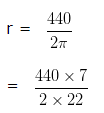= 70cm
Hence, the radius of the circle  = 70cm

Correct Answer – a) 70 cm

Area and Perimeter Worksheets Grade 6 – Explanation 13-

Let, the radius of the given circle be r cm
As we know that,
Circumference of the circle = 2∏r cm
Given,
Circumference of a cicle = 44cm
Therefore,
2∏r = 44 (from above)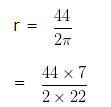= 7cm
Diameter of the circle = 2r
= (2 x 7)cm
= 14cm
Hence, the Diameter of the circle is 14 cm

Correct Answer – c) 14 cm

Area and Perimeter Worksheets Grade 6 – Explanation 14-

Length of the field = 80 m
Breadth of the field = 60 m
Perimeter of the field = 2 (l+b) units
= 2 ( 80 + 60 ) m
= ( 2 x 140 ) m
= 280 m
Total length of rope = 3 x Perimeter of the field
= ( 3 x 280 ) m
= 840 m

Correct Answer – a) 840 m

Area and Perimeter Worksheets Grade 6 – Explanation 15-

Radius of the wheel = 140/2 cm = 70 cm

Circumference of the wheel = 2∏r
= ( 2 x 22/7 x 70 ) cm

= 440 cm
= 4.4 m ( As we know that, 1 m = 100 cm )
Distance covered by wheel in 1 revolution = Circumference of the wheel
Distance covered by wheel in 1 revolution = 4.4 m
Distance covered by wheel in 1000 revolutions = ( 4.4 x 1000 ) m
= 4400 m
= 4.4 km ( As we know that, 1 m = 1000 km )
Hence, Distance covered by wheel in 1000 revolutions is 4.4 km

Correct Answer – d) 4.4 km

Area and Perimeter Worksheets Grade 6 – Explanation 16-

Length of the room = 125 m
Breadth of the room = 10 m
Area of the floor of the room = ( 125 x 10 ) m²
= 1250 m²
Area of carpet required = 1250 m²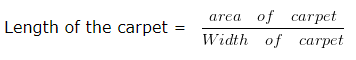= 1250 m²/5 m = 1250 m/5 = 250 m
Rate of carpeting = 20 per metre.
Cost of carpeting = ₹ ( 250 x 20 ) = ₹ 5000

Correct Answer – b) ₹ 5000

Area and Perimeter Worksheets Grade 6 – Explanation 17-

Let, length and breadth be 3y m and 5y m
Since, perimeter of rectangle = 2 (l+b) units
= 2 ( 3 y + 5 y ) m
= ( 2 x 8 y ) m
= 16ym
But, perimeter of rectangle = 32 m
Since, 16 y = 32
y = 32/16
y = 2
So, length = ( 3 x 2 ) m = 6 m
breadth = ( 5 x 2 ) m = 10 m

Correct Answer – c) Length = 6 m , breadth = 10 m

Area and Perimeter Worksheets Grade 6 – Explanation 18-

Length of the field = 65 m
Breadth of the field = 55 m
Perimeter of the field = 2 ( l+b ) units
= 2 ( 65 + 55 ) m
= ( 2 x 120 ) m
= 240 m
Cost of fencing per metre = ₹ 10
Total cost of fencing = ₹ ( 240 x 10 )
= ₹ 2400

Correct Answer – b) ₹ 2400

Area and Perimeter Worksheets Grade 6 – Explanation 19-

Given
Area = 600 cm²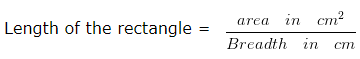= 600 cm²/30 cm = 600 cm/30 = 20 cm
Perimeter of the rectangle = 2 x ( l + b ) cm
= 2 x ( 30 + 20 ) cm
= ( 2 x 50 ) cm
= 100 cm
Hence, the perimeter of the rectangle is 100 cm

Correct Answer – b) 100 cm

Area and Perimeter Worksheets Grade 6 – Explanation 20-

Given
Area = 108 cm²
length = 12 cm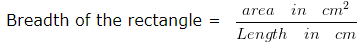= 108 cm²/12 cm = 108 cm/12 = 9 cm
Perimeter of the rectangle = 2 x ( l + b ) cm
= 2 x ( 12 + 9 ) cm
= ( 2 x 21 ) cm
= 42 cm
Hence, perimeter of the rectangle is 42 cm

Correct Answer – b) 42 cm